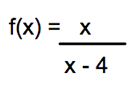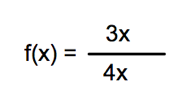# Graphing Rational Functions That Have Linear Polynomials: Steps & Examples

Instructions:

Choose an answer and hit 'next'. You will receive your score and answers at the end.

question 1 of 3

### What is the vertical asymptote of the following function?Create Your Account To Take This Quiz

As a member, you'll also get unlimited access to over 75,000 lessons in math, English, science, history, and more. Plus, get practice tests, quizzes, and personalized coaching to help you succeed.

Try it risk-free for 30 days. Cancel anytime.

### 1. What is the horizontal asymptote for the following function?### 2. Which of the following is a possible function for this graph?Create your account to access this entire worksheet
A Premium account gives you access to all lesson, practice exams, quizzes & worksheets
Quizzes, practice exams & worksheets
Certificate of Completion
Create an account to get started

The quiz is a collection of math problems. These questions will ask you to glean information about a provided function. Some of the other questions will give you a property of a particular function and ask you to identify that function.

## Quiz & Worksheet Goals

The assessment will help you go over the following topics:

• Rational functions
• Vertical asymptotes
• Linear polynomials
• Horizontal asymptotes

## Skills Practiced

The quiz will help you go over these skills:

• Reading comprehension - ensure that you draw the most important information from the related linear polynomials lesson
• Making connections - use understanding of the concept of linear polynomials
• Critical thinking - apply relevant concepts to examine information about linear polynomials in a different light
• Problem solving - use acquired knowledge to solve rational function practice problems

Finish the quiz and then head over to the accompanying lesson Graphing Rational Functions That Have Linear Polynomials: Steps & Examples. The lesson will help you cover the following information:

• Defining rational functions
• Exploring linear polynomials
• Describing vertical asymptotes
• Detailing horizontal asymptotes
• Understanding graphs of horizontal and vertical asymptotes
Final ExamMath 105: Precalculus Algebra
Status: Not Started
Chapter ExamRational Expressions and Functions
Status: Not Started

Support